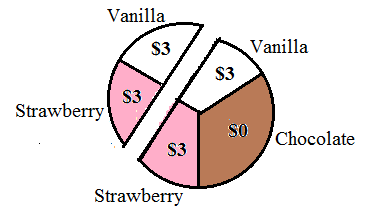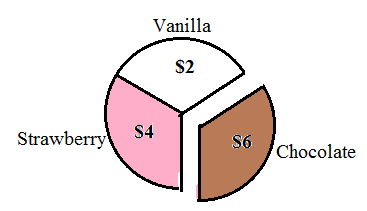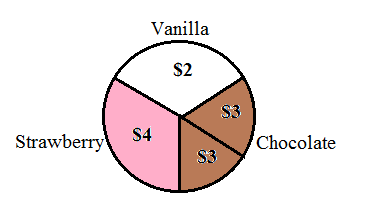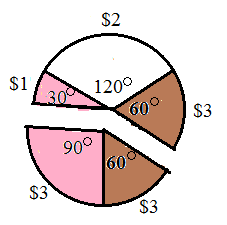$$\newcommand{\id}{\mathrm{id}}$$ $$\newcommand{\Span}{\mathrm{span}}$$ $$\newcommand{\kernel}{\mathrm{null}\,}$$ $$\newcommand{\range}{\mathrm{range}\,}$$ $$\newcommand{\RealPart}{\mathrm{Re}}$$ $$\newcommand{\ImaginaryPart}{\mathrm{Im}}$$ $$\newcommand{\Argument}{\mathrm{Arg}}$$ $$\newcommand{\norm}{\| #1 \|}$$ $$\newcommand{\inner}{\langle #1, #2 \rangle}$$ $$\newcommand{\Span}{\mathrm{span}}$$

8.1: Basic Concepts of Fair Division

•• Maxie Inigo, Jennifer Jameson, Kathryn Kozak, Maya Lanzetta, & Kim Sonier
• Faculty (Mathematics) at Coconino Community College
$$\newcommand{\vecs}{\overset { \rightharpoonup} {\mathbf{#1}} }$$ $$\newcommand{\vecd}{\overset{-\!-\!\rightharpoonup}{\vphantom{a}\smash {#1}}}$$$$\newcommand{\id}{\mathrm{id}}$$ $$\newcommand{\Span}{\mathrm{span}}$$ $$\newcommand{\kernel}{\mathrm{null}\,}$$ $$\newcommand{\range}{\mathrm{range}\,}$$ $$\newcommand{\RealPart}{\mathrm{Re}}$$ $$\newcommand{\ImaginaryPart}{\mathrm{Im}}$$ $$\newcommand{\Argument}{\mathrm{Arg}}$$ $$\newcommand{\norm}{\| #1 \|}$$ $$\newcommand{\inner}{\langle #1, #2 \rangle}$$ $$\newcommand{\Span}{\mathrm{span}}$$ $$\newcommand{\id}{\mathrm{id}}$$ $$\newcommand{\Span}{\mathrm{span}}$$ $$\newcommand{\kernel}{\mathrm{null}\,}$$ $$\newcommand{\range}{\mathrm{range}\,}$$ $$\newcommand{\RealPart}{\mathrm{Re}}$$ $$\newcommand{\ImaginaryPart}{\mathrm{Im}}$$ $$\newcommand{\Argument}{\mathrm{Arg}}$$ $$\newcommand{\norm}{\| #1 \|}$$ $$\newcommand{\inner}{\langle #1, #2 \rangle}$$ $$\newcommand{\Span}{\mathrm{span}}$$

How do we divide items or collections of items among 2 or more people so that every person feels he/she received a fair share: Different people may assign a different value to the same item. A “fair share” to one person may not be the same as a “fair share” to another person. The methods in this chapter will guarantee that everyone gets a “fair share” but it might not be the “fair share” he/she wanted.

People often refer to fair division as a game. It has players and rules just like a game. The set of goods to be divided is called S. The players P1, P2, P3,…, Pn are the parties entitled to a share of S. Each player must be able to assign a value to the set S or any subset of the set S.

In a continuous fair-division game the set S is divisible in an infinite number of ways, and shares can be increased or decreased by arbitrarily small amounts. Typical examples of continuous fair-division games involve the division of land, cake, pizza, etc… A fair-division game is discrete when the set S is made up of objects that are indivisible like paintings, houses, cars, boats, jewelry, etc. A pizza can be cut into slices of almost any size but a painting cannot be cut into pieces. To make the problems simple to think about, we will use cakes or pizzas for continuous examples and collections of small candies for discrete examples. A mixed fair-division game is one in which some of the components are continuous and some are discrete and is not covered in this book.

The method we use to divide a cake or pizza can be used to divide a piece of land or to divide the rights of access to mine the ocean floor (between countries). The method we use to divide a mixed bag of Halloween candy can be used to divide a large jewelry collection. This book will not get to all of them but we can also use fair division ideas to decide which transplant patient gets a liver when it becomes available, or to help two companies merge (who is CEO, who has layoffs, which name to use, etc.).

Rules: In order for the division of S to be fair, the players in the game must be willing participants and accept the rules of the game as binding.

• The players must act rationally according to their system of beliefs.
• The rules of mathematics apply when assigning values to the objects in S.
• Only the players are involved in the game, there are no outsiders such as lawyers.

If the players follow the rules the game will end after a finite number of moves by the players and result in a division of S.

Assumptions: We must assume the following:

• The players all play fair.
• They have no prior information about the likes or dislikes of the other players.
• They do not assign values in a way to manipulate the game.
• All players have equal rights in sharing the set S. In other words, if there are three players, each player is entitled to at least 1/3 of S.

If these assumptions are not met, the division may not be totally fair.

What is a fair share?

The basic idea in a continuous fair division game is that S is divided into pieces. Each player assigns a value to each piece of S. Based on these values a player decides which pieces he/she considers a fair share. Since each player is entitled to at least a proportional share of S, it is easy to determine what is considered a fair share.

Example $$\PageIndex{1}$$: Determining Fair Shares

Four players Abby, Betty, Christy, and Debbie are to divide a cake S. The cake has been sliced into four pieces, S1, S2, S3, and S4 (not necessarily the same size or with the same amount of frosting). Each of the players has assigned a value to each piece of cake as shown in the following table.

Table $$\PageIndex{1}$$: Values of Each Piece of Cake

Player/Piece

S1

S2

S3

S4

Abby

10%

50%

30%

10%

Betty

30%

30%

10%

30%

Christy

40%

20%

20%

20%

Debbie

25%

25%

25%

25%

Which of the pieces would each player consider a fair share?

Since there are four players, each player is entitled to at leastof S.

The fair shares are highlighted in the following table.

Table $$\PageIndex{2}$$: Fair Shares for Each Player

Player/Piece

S1

S2

S3

S4

Abby

10%

50%

30%

10%

Betty

30%

30%

10%

30%

Christy

40%

20%

20%

20%

Debbie

25%

25%

25%

25%

Find the Value of a Piece of S

How did the players in Example $$\PageIndex{1}$$ determine the value of each piece: Part of the value is emotional and part is mathematical. Consider three heirs who must split up an island consisting of a stretch of beach, a small mountain, and a large meadow. A person who really likes the beach may be willing to settle for a smaller piece of the island to get the beach.

For the examples in this chapter, all cakes will be drawn in two-dimensions. The height of the cake is not relevant to the problem as long as the cake is uniform in height.

It will be helpful to remember that there are 360◦ in a full circle.

Example $$\PageIndex{2}$$: The Value of a Slice of S, #1

Fred buys a half strawberry, half vanilla cake for $9. He loves vanilla cake but only likes strawberry cake so he values the vanilla half of the cake twice as much as the strawberry cake. Find the values of slices S1, S2, and S3 in the following figure.Figure $$\PageIndex{3}$$: Half Strawberry/Half Vanilla Cake with Three Slices Find the value of each half of the cake using algebra. Let x = the value of the strawberry half of the cake. Then 2x = the value of the vanilla half of the cake. The total value of the cake is$9.

\begin{align*} x+2 x &= \ 9 \\[4pt] 3 x &=\ 9 \\[4pt] x &=\ 3 \end{align*}

The strawberry half is worth $3 and the vanilla half is worth$6.

1. Value of S1:

$$60^{\circ}$$ is 1/3 of $$180^{\circ}$$ (the strawberry half of the cake) so the slice is worth 1/3 of $3. $\frac{60^{\circ}}{180^{\circ}}(\ 3)=\ 1$ 1. Value of S2: 45◦ is 1/4 of 180◦ (the vanilla half of the cake) so the slice is worth 1/4 of$6.

$\frac{45^{\circ}}{180^{\circ}}(\ 6)=\ 1.50$

1. Value of S3:

Since S3 is part strawberry and part vanilla we must find the value of each part separately and then add them together.

$\frac{30^{\circ}}{180^{\circ}}(\ 3)+\frac{40^{\circ}}{180^{\circ}}(\ 6)=\ 1.83$

Example $$\PageIndex{3}$$: The Value of a Slice of S, #2

Tom and Fred were given a cake worth $12 that is equal parts strawberry, vanilla and chocolate. Tom likes vanilla and strawberry the same but does not like chocolate at all. Fred will eat vanilla but likes strawberry twice as much as vanilla and likes chocolate three times as much as vanilla.Figure $$\PageIndex{4}$$: Three-Flavored Cake Solution 1. How should Tom divide the cake into two pieces so that each piece is a fair share to him? The cake is worth$12 so a fair share is. Tom must cut the cake into two pieces. They do not have to be the same size but they do have to have the same value of $6 in Tom’s eyes. Tom does not like chocolate so all the value of the cake is in the strawberry and vanilla parts. Also, Tom likes vanilla and strawberry equally well so each of these parts of the cakes are worth$6 in his eyes.Figure $$\PageIndex{5}$$: How Tom Sees the Cake

The easiest division is to cut the cake so that one slice contains all the strawberry part and half the chocolate part and the other slice contains all the vanilla part and the other half of the chocolate part.Figure $$\PageIndex{6}$$: Tom’s Two Pieces

Note that Tom could slice the cake along a chord of the circle as shown below. The mathematics involved in that type of cut is beyond the scope of this courseFigure $$\PageIndex{7}$$: Another Possibility for Tom’s Two Pieces
1. How should Fred divide the cake into two pieces so that each piece is a fair share to him?

Let x = value of the vanilla part to Fred.

Then 2x = value of the strawberry part.

Also, 3x = value of the chocolate part.

\begin{align*} x+2 x+3 x &=12 \\ 6 x&=12 \\ x&=2 \\ 2 x&=4 \\ 3 x&=6 \end{align*}Figure $$\PageIndex{8}$$: How Fred Sees the Cake

Fred needs to cut the cake into two pieces so that each piece has a value of $6. An obvious choice would be to make the chocolate part one of the pieces and both the vanilla and strawberry parts the other piece. As you can see in Figure $$\PageIndex{9}$$ each of the pieces is worth a total of$6.Figure $$\PageIndex{9}$$: First Possibility for Fred’s Two Pieces
1. Find another way for Fred to divide the cake.

There are many possibilities. Let’s assume he wants to cut the chocolate part in half since he likes chocolate the most. This will guarantee that Fred gets some of the chocolate part regardless of which piece he gets in the game. The chocolate part is worth $6 to Fred so each half of the chocolate piece would be worth$3.Figure $$\PageIndex{10}$$: Dividing the Chocolate Part in Half

Fred needs to add another $3 worth of cake to each of the chocolate pieces. It is easy to see that Fred needs to cut the cake somewhere in the strawberry part so that the strawberry part is cut into two pieces. Let’s call the smaller piece of the strawberry part “piece 1” and the larger piece of the strawberry part “piece 2.” Piece 1 needs to be worth$1 and piece 2 needs to be worth $3 so that each slice of the cake ends up with a total of$6.Figure $$\PageIndex{11}$$: Dividing the Strawberry and Vanilla Parts

The strawberry part of the whole cake is 1/3 of the cake or $$\frac{1}{3}\left(360^{\circ}\right)=120^{\circ}$$. Piece 2 is worth $3 of the$4 for the strawberry part so it is ¾ of the 120◦ or 90◦. Piece 1 would be 120◦ - 90◦ = 30◦.Figure $$\PageIndex{12}$$: Second Possibility for Fred’s Two Pieces
Example $$\PageIndex{4}$$: The Value of a Slice of S, #3

George and Ted wish to split a 12-inch sandwich worth $9. Half the sandwich is vegetarian and half the sandwich is meatball. George does not eat meat at all. Ted likes the meatball part twice as much as vegetarian part.Figure $$\PageIndex{13}$$: Sub Sandwich 1. How should George divide the sandwich so that each piece is a fair share to him? George is a vegetarian so all the value is in the vegetarian half of the sandwich. He should divide the vegetarian part of the sandwich in half. One piece will be all of the meatball part plus three inches of the vegetarian part. The second piece will just be three inches of the vegetarian part.Figure $$\PageIndex{14}$$: How George Should Cut the Sandwich 1. How should Ted divide the sandwich so that each piece is a fair share to him? Let x be the value of vegetarian part of the sandwich. Then $$2x$$ is the value of the meatball part of the sandwich.Ted sees the meatball part with a value of$6 and the vegetarian part with a value of $3. A fair share to Ted would be$4.50, half the value of the sandwich. Ted must divide the sandwich somewhere in the meatball part.

$\frac{\ 4.50}{\ 6.00}=\frac{3}{4}, \quad \frac{3}{4}(6 ")=4 \frac{1}{2} \text { inches }$Figure $$\PageIndex{15}$$: How Ted Should Cut the Sandwich